11 Basic Geospatial EDA

In the previous chapters, you were introduced to the basics of "dplyr" and "ggplot2", performing various operations on the data storms. Because this data set contains geographical information such as longitude and latitude, we take a further step in this chapter in order to learn about plotting basic geographical maps.

You will need the following packages:

library(dplyr)    # for syntactic manipulation of tables
library(ggplot2)  # for making graphs based on tabular data
library(maps)     # for drawing basic geographical maps
library(rnaturalearth)   # world map data from Natural Earth

and the following objects:

storms75 <- filter(storms, year == 1975)

11.1 Graphing Maps

In this chapter, we give a basic exposure to plotting maps with "ggplot2", jointly with "maps". Keep in mind that there is a wide array of packages for graphing all sorts of maps, and geospatial information. Good resources to look at are:

11.1.1 Plotting location of storm records

For illustration purposes, we continue using the data frame storms75. Having latitude and longitude, we can make a scatterplot to see the location of the storm records. Recall that the ggplot function to do this is geom_point():

ggplot(data = storms75, aes(x = long, y = lat)) +
geom_point()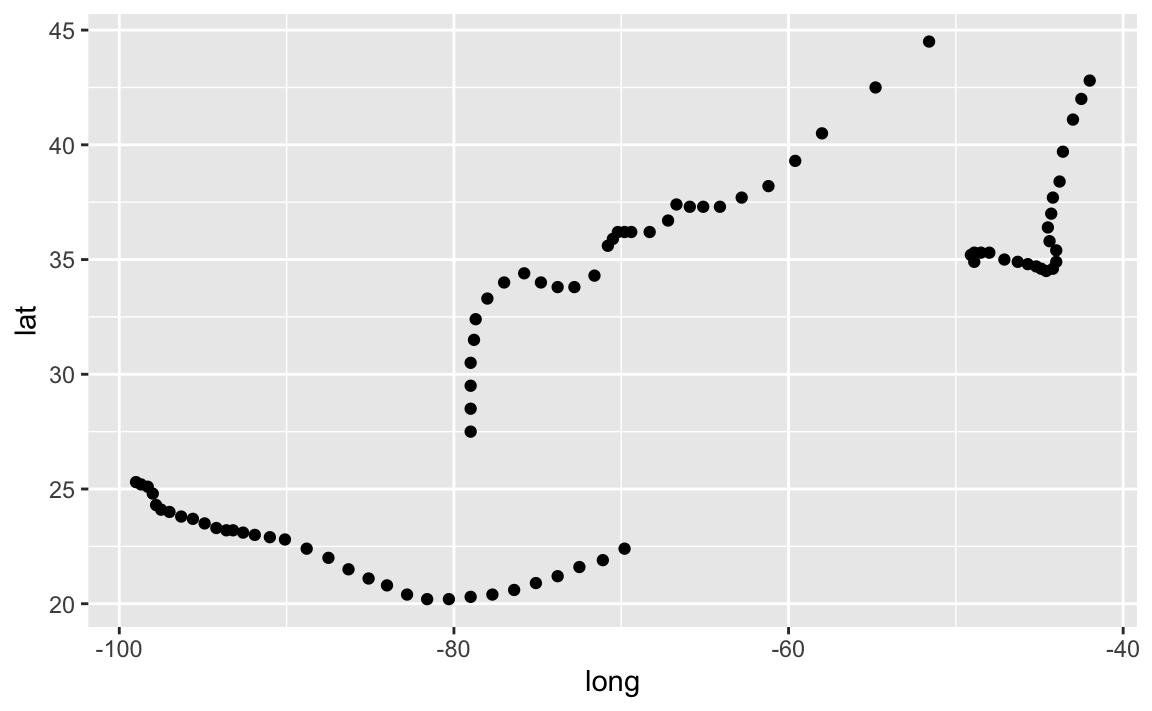Each dot represents the location of a single storm record, with longitude in the x-axis, and latitude in y-axis. As you can tell from the previous plot, there are three sets of dotted-patterns, which as you may expect, correspond to the three storms that occurred in 1975. To distinguish each storm, we can color the dots by taking into account the different storm names. This involves mapping the column name to the color attribute:

ggplot(data = storms75, aes(x = long, y = lat, color = name)) +
geom_point()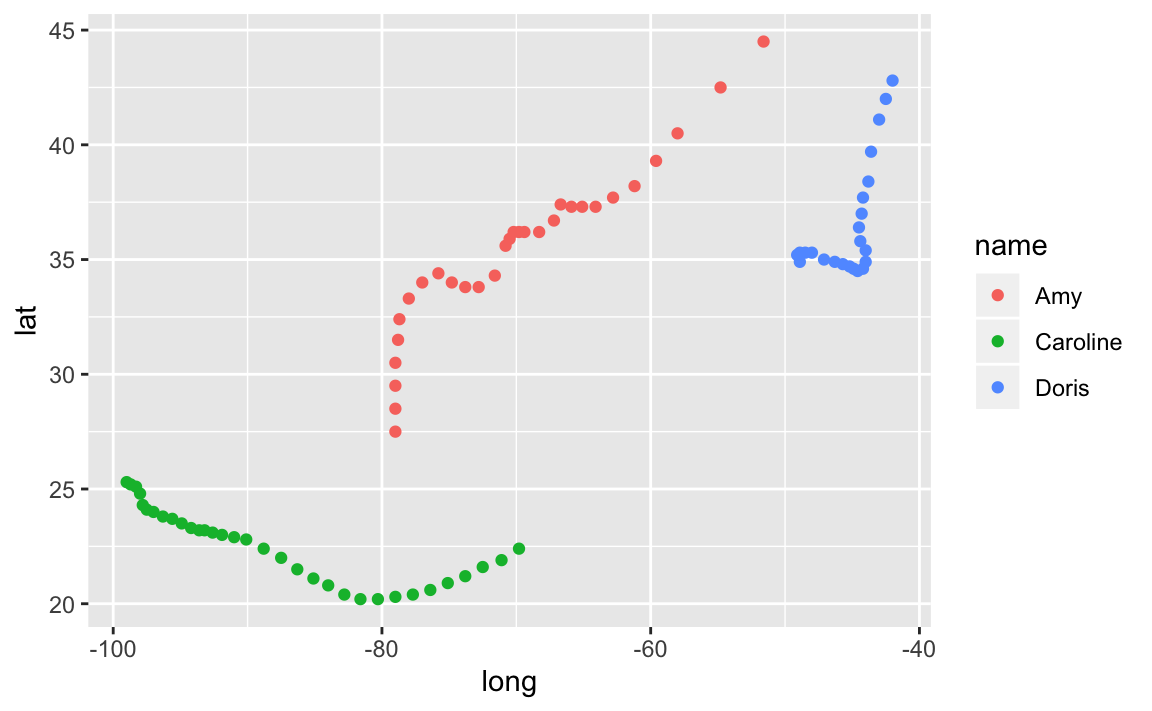Keep in mind that the previous command can also be written as:

# alternative ways to write equivalent commands
ggplot(data = storms75) +
geom_point(aes(x = long, y = lat, color = name))

ggplot() +
geom_point(data = storms75, aes(x = long, y = lat, color = name))

The above scatterplot is a good starting point to visualize the location of the storm records, but it would be nice to have an actual image of a map. Let’s see how to do this in the following subsections.

11.1.2 Basic maps with ggplot2

One of the simplest and oldest ways to plot maps in R is with the package "maps". Nowadays, there are more sophisticated packages for geospatial data and making maps, but let’s not worry about them at this moment. The "maps" package provides the map() function that lets you plot basic geographical maps. One of such maps is a world map, which can easily be plotted with this command:

map("world")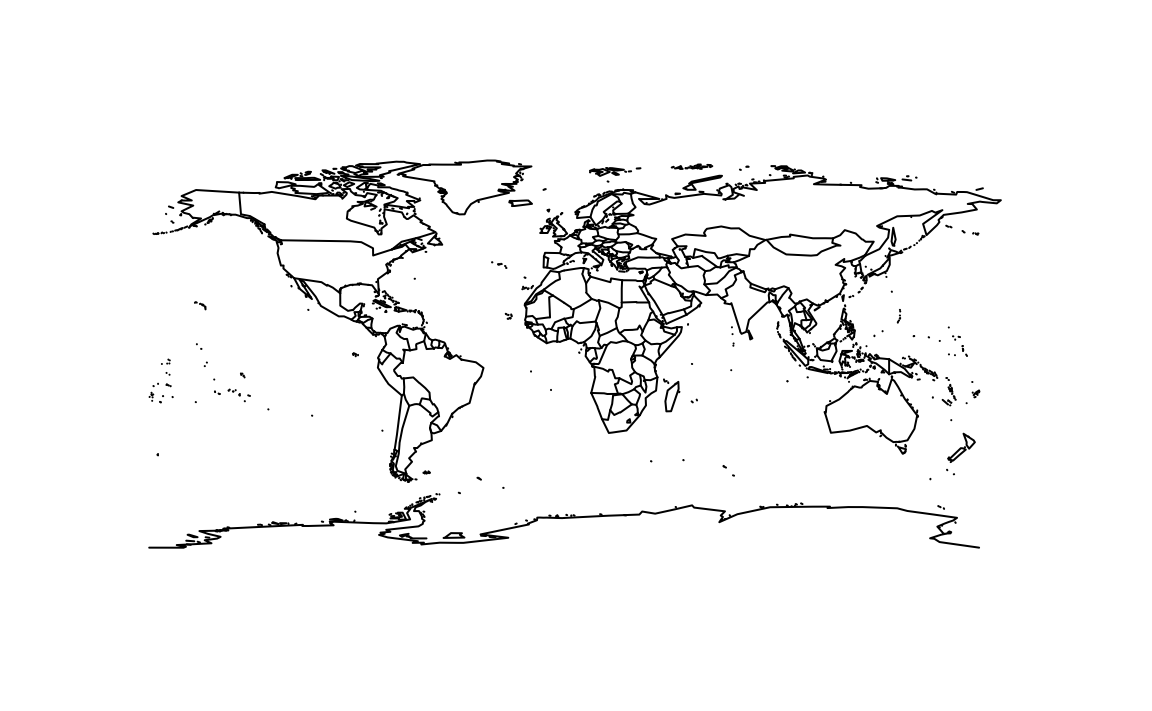In order to use any of the map data objects from "maps" with "ggplot2", you first need to convert the map-object into a data frame. This is done with the "ggplot2" function map_data(). This function takes in the name of a map, and it retrieves the map data—from "maps"—into a data.frame:

# get world map data frame
world_map <- map_data("world")

#>    long  lat group order region subregion
#> 1 -69.9 12.5     1     1  Aruba      <NA>
#> 2 -69.9 12.4     1     2  Aruba      <NA>
#> 3 -69.9 12.4     1     3  Aruba      <NA>
#> 4 -70.0 12.5     1     4  Aruba      <NA>
#> 5 -70.1 12.5     1     5  Aruba      <NA>
#> 6 -70.1 12.6     1     6  Aruba      <NA>

Notice that world_map contains longitude and latitude columns, as well as group which is a grouping variable for each of the map polygons. Once you have the world_map data frame, you can plot it with the geometric polygon function geom_polygon().

# basic world map with ggplot()
ggplot() +
geom_polygon(data = world_map, aes(x = long, y = lat, group = group)) +
theme_bw()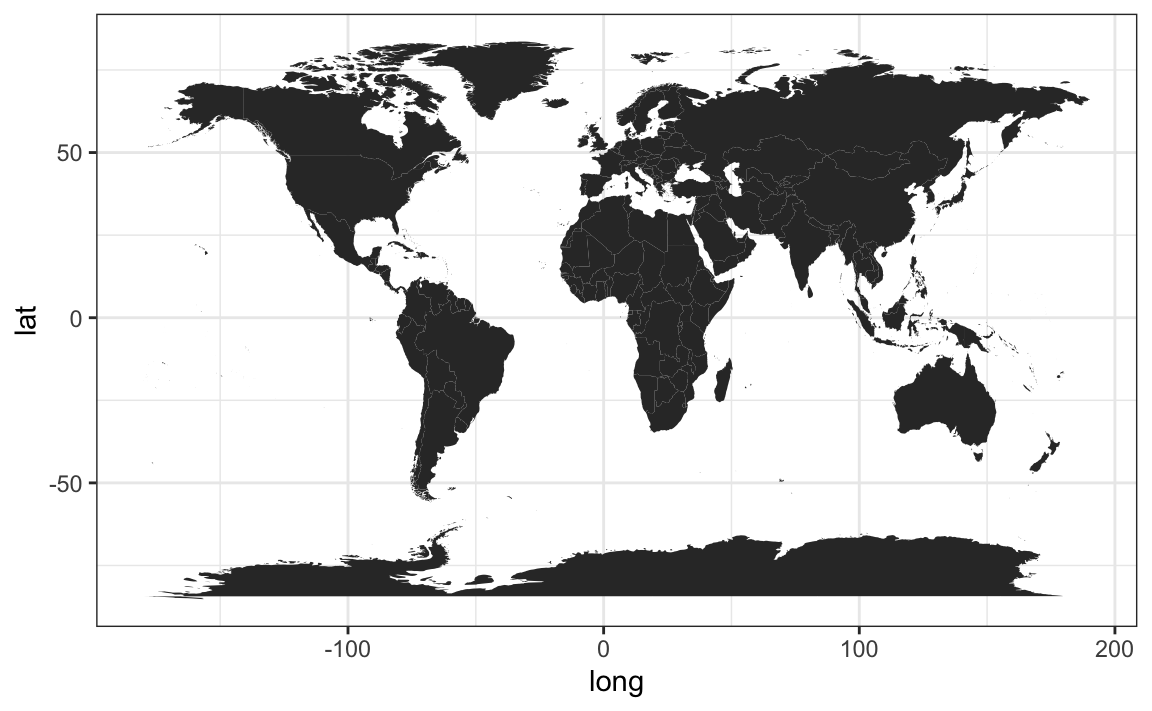In the above command, notice how we specify the data argument inside geom_polygon() instead of inside ggplot(). We do this because the data frame world_map is used to graph the polygons layer of the map. Also, because we are plotting a map, we simplify the background theme to black-and-white—theme_bw().

To handle the code more easily, we create a "ggplot" object called gg_world. We’ll use this object as our “canvas” for plotting the storm locations.

# map "canvas" stored as gg_world
gg_world <- ggplot() +
geom_polygon(data = world_map,
aes(x = long, y = lat, group = group),
fill = "gray95", colour = "gray70", size = 0.2) +
theme_bw()

gg_world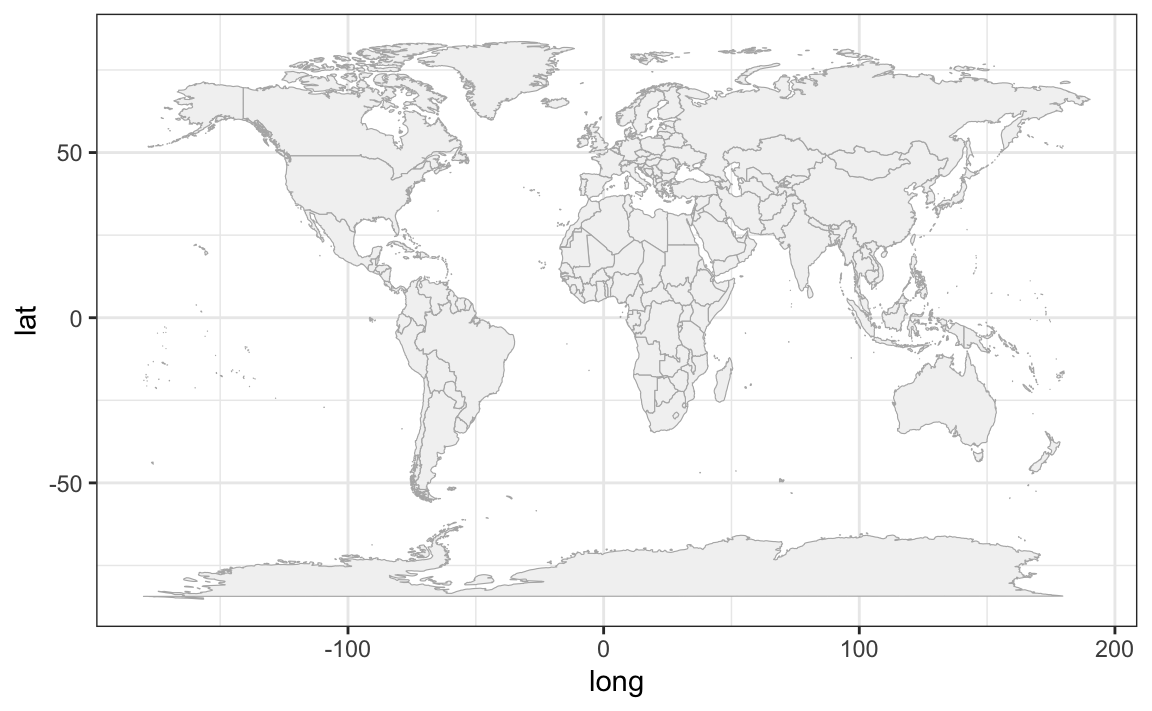Now that we know how to plot a map with ggplot(), we can add the points of the storm records. This is done with geom_point(), and specifying storms75 as the data argument inside this function. In other words, we are using two separate data frames. One is world_map, used to draw the polygons of the map; the other one is stomrs75 to graph the dots of each storm. Notice also that there are no inputs provided to the function ggplot().

# world map, adding storms in 1975
gg_world +
geom_point(data = storms75, aes(x = long, y = lat, color = name))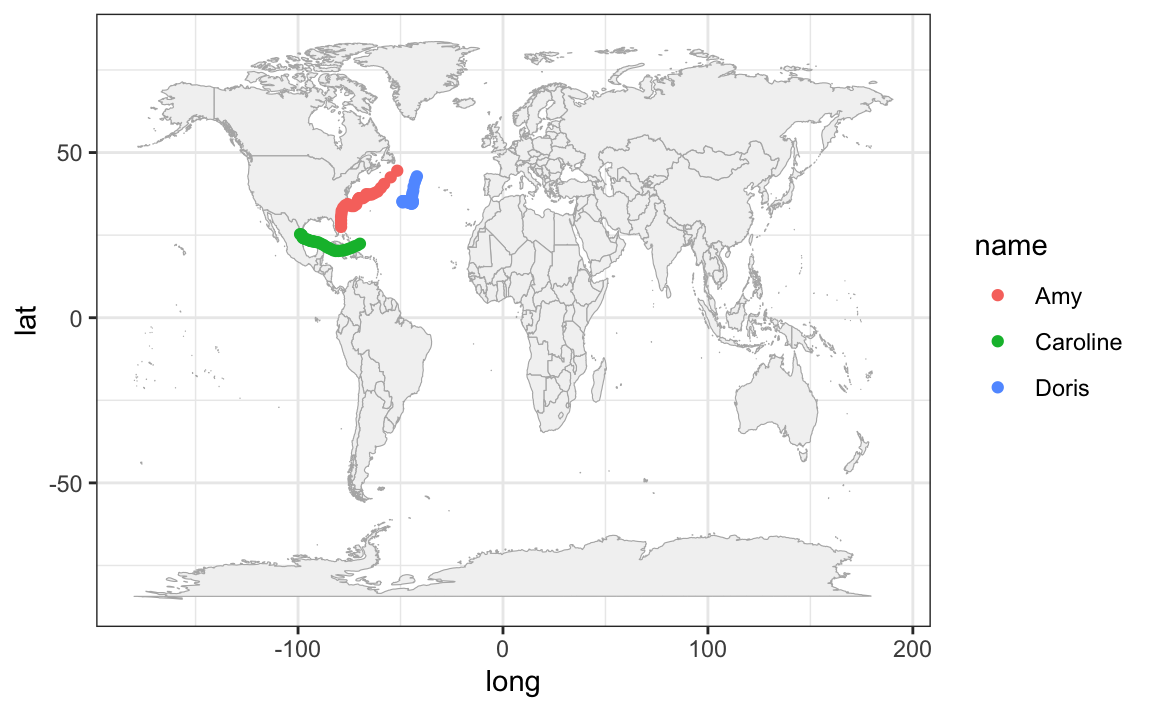Because the analyzed hurricanes occurred in the North Atlantic basin, we can focus on that region by modifying the x-and-y axis limits:

# zoom-in (North Atlantic)
gg_world +
geom_point(data = storms75,
aes(x = long, y = lat, color = name)) +
xlim(c(-150, 0)) +
ylim(c(0, 90))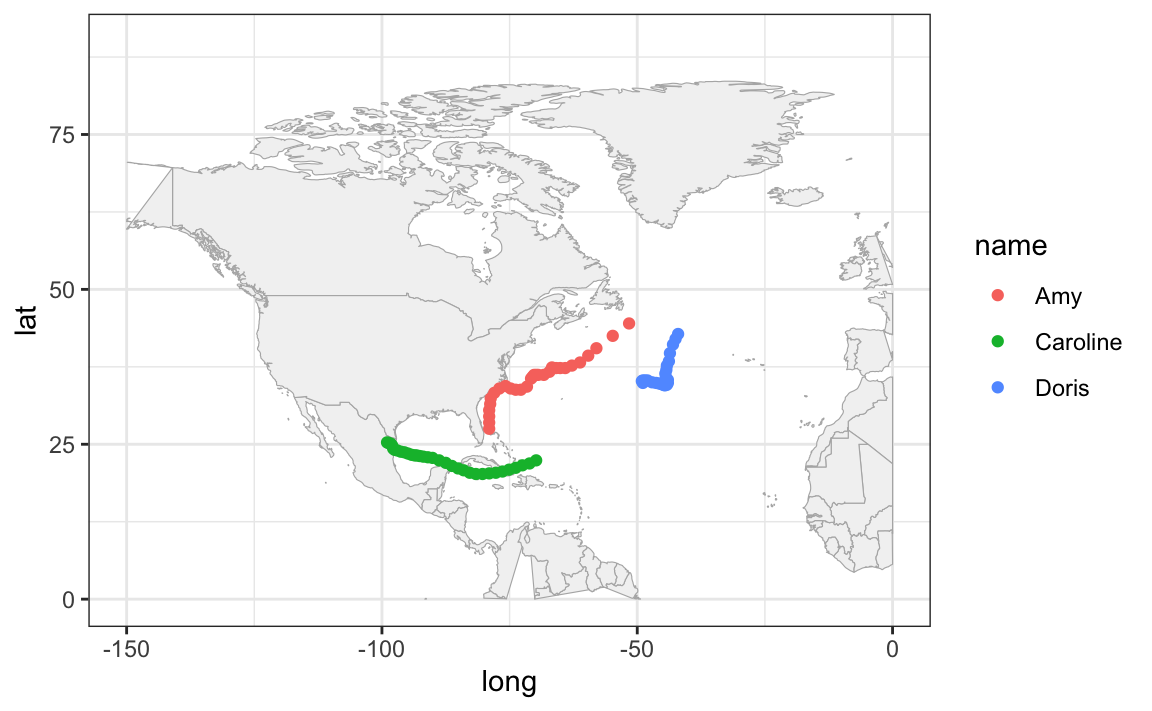It’s worth mentioning that this zooming-in has a secondary effect of distorting some of the polygons. For example, Alaska seems to get cut in half. Also the polygon of Colombia is incomplete. Ignoring these distortions for now, we can continue exploring things by taking into account more variables. For instance, let’s map the wind speed to the size argument of points.

# using wind values to change the size of dots
gg_world +
geom_point(data = storms75,
aes(x = long, y = lat, color = name, size = wind),
alpha = 0.5) +
xlim(c(-150, 0)) +
ylim(c(0, 90))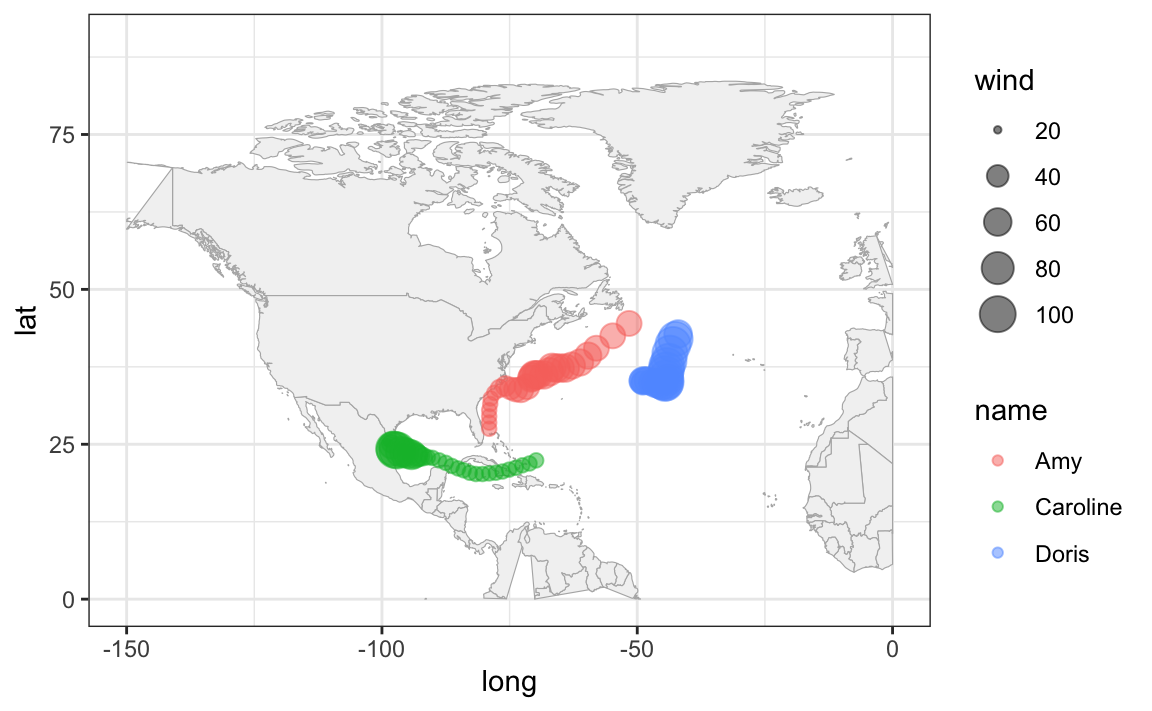A very similar appearance can be achieved by replacing geom_point() with geom_path():

gg_world +
geom_path(data = storms75,
aes(x = long, y = lat, color = name, size = wind),
lineend = "round", alpha = 0.4) +
xlim(c(-150, 0)) +
ylim(c(0, 90))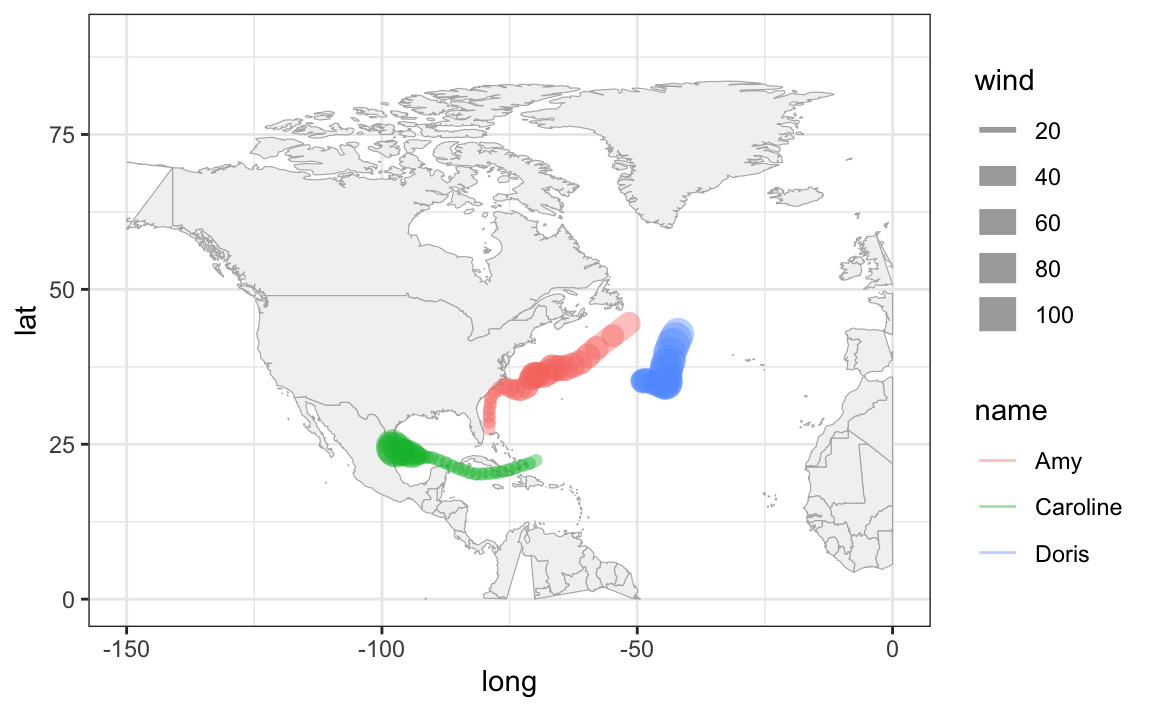11.1.3 More mapping approaches

Another interesting map graphing approach is by using map-objects from the package "rnaturalearth".

We use the ne_countries() function—from "rnaturalearth"—to get world country polygons. In the following command, we specify a medium scale resolution, and a returned object of class "sf" (simple features).

# another world data frame
world_df <- ne_countries(scale = "medium", returnclass = "sf")
class(world_df)
#>  "sf"         "data.frame"

Now we can pass world_df to ggplot(), and use geom_sf() which is the function that allows us to visualize simple features objects "sf".

# another world map (from "rnaturalearth")
ggplot(data = world_df) +
geom_sf() +
theme_bw()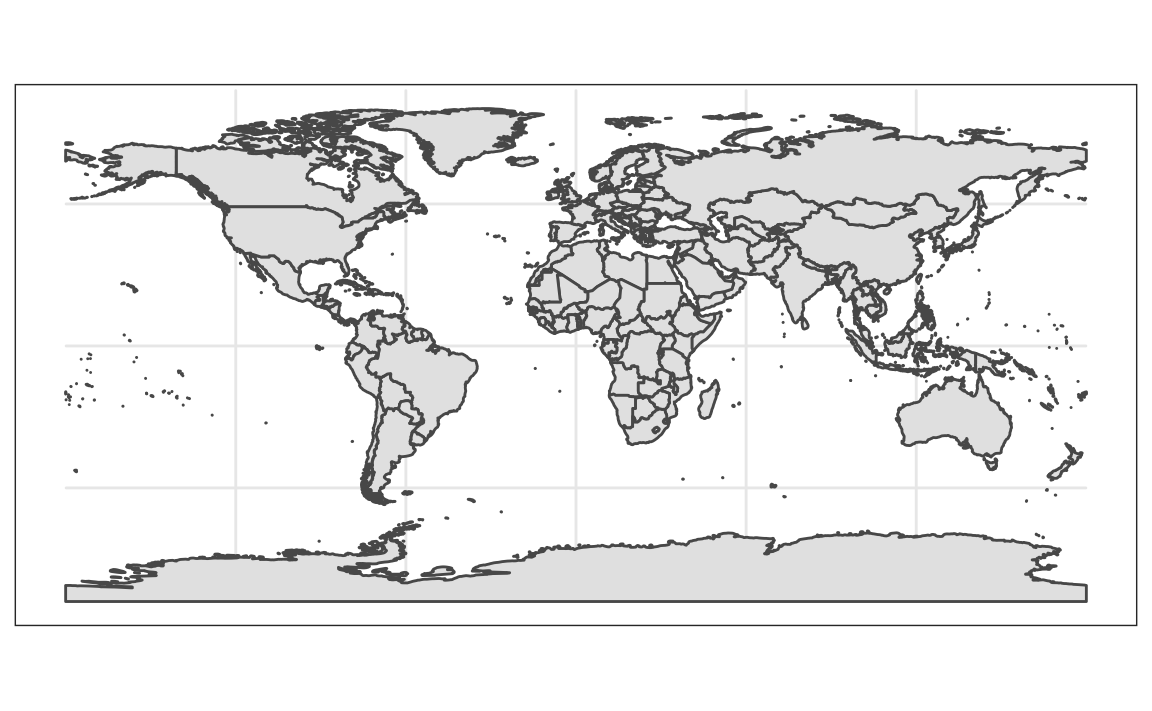One advantage of using this other mapping approach is that we can zoom-in without having distorted polygons. To focus on a specfic region, we set the x-axis and y-axis limits with the coord_sf() function. Again, for coding convenience, let’s create another "ggplot" object

# ggplot object to be used as a canvas
gg_world2 <- ggplot(data = world_df) +
geom_sf() +
coord_sf(xlim = c(-150, 0), ylim = c(0, 90), expand = TRUE) +
theme_bw()

gg_world2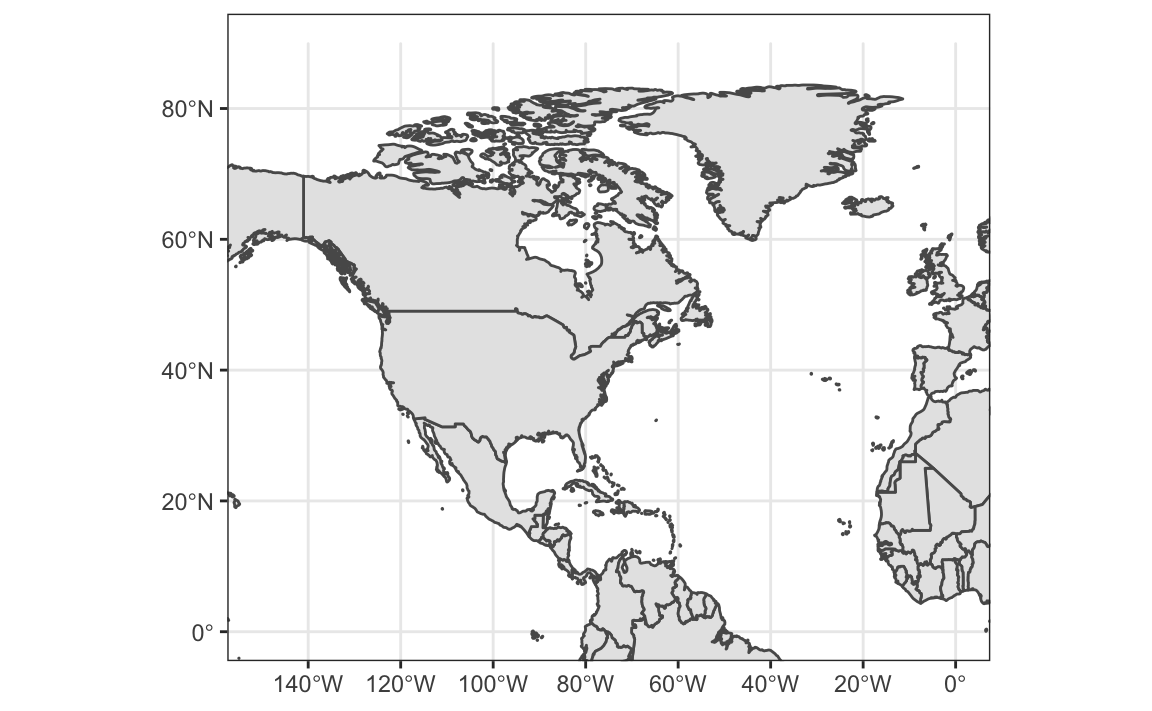gg_world2 +
geom_path(data = storms75,
aes(x = long, y = lat, color = name),
lineend = "round", size = 2, alpha = 0.8)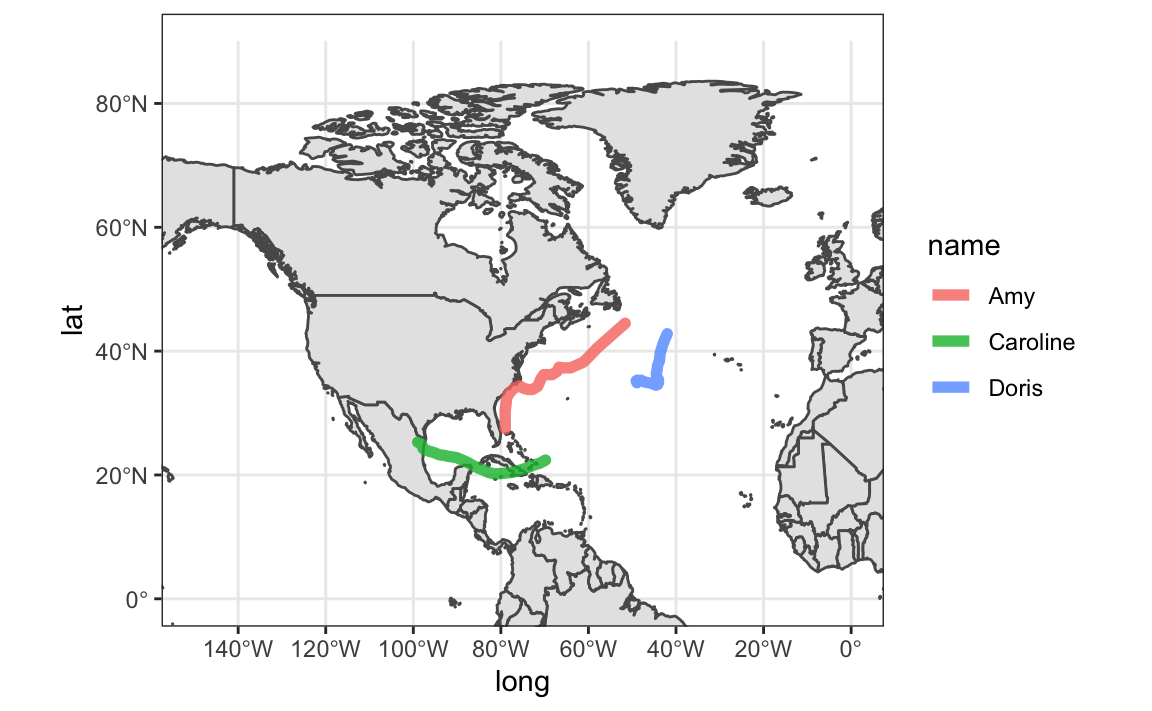11.1.4 Storms from 1975 to 1980

As a simple experiment, let’s graph storms between 1975 and 1980 (six years). First we create a dedicated data table storms_75_80 to select the rows we are interested in:

storms_75_80 <- filter(storms, year %in% 1975:1980)

And then we can use facet_wrap(~ year) to graph storms by year:

gg_world +
geom_path(data = storms_75_80,
aes(x = long, y = lat, group = name),
lineend = "round") +
xlim(c(-150, 0)) +
ylim(c(0, 90)) +
facet_wrap(~ year)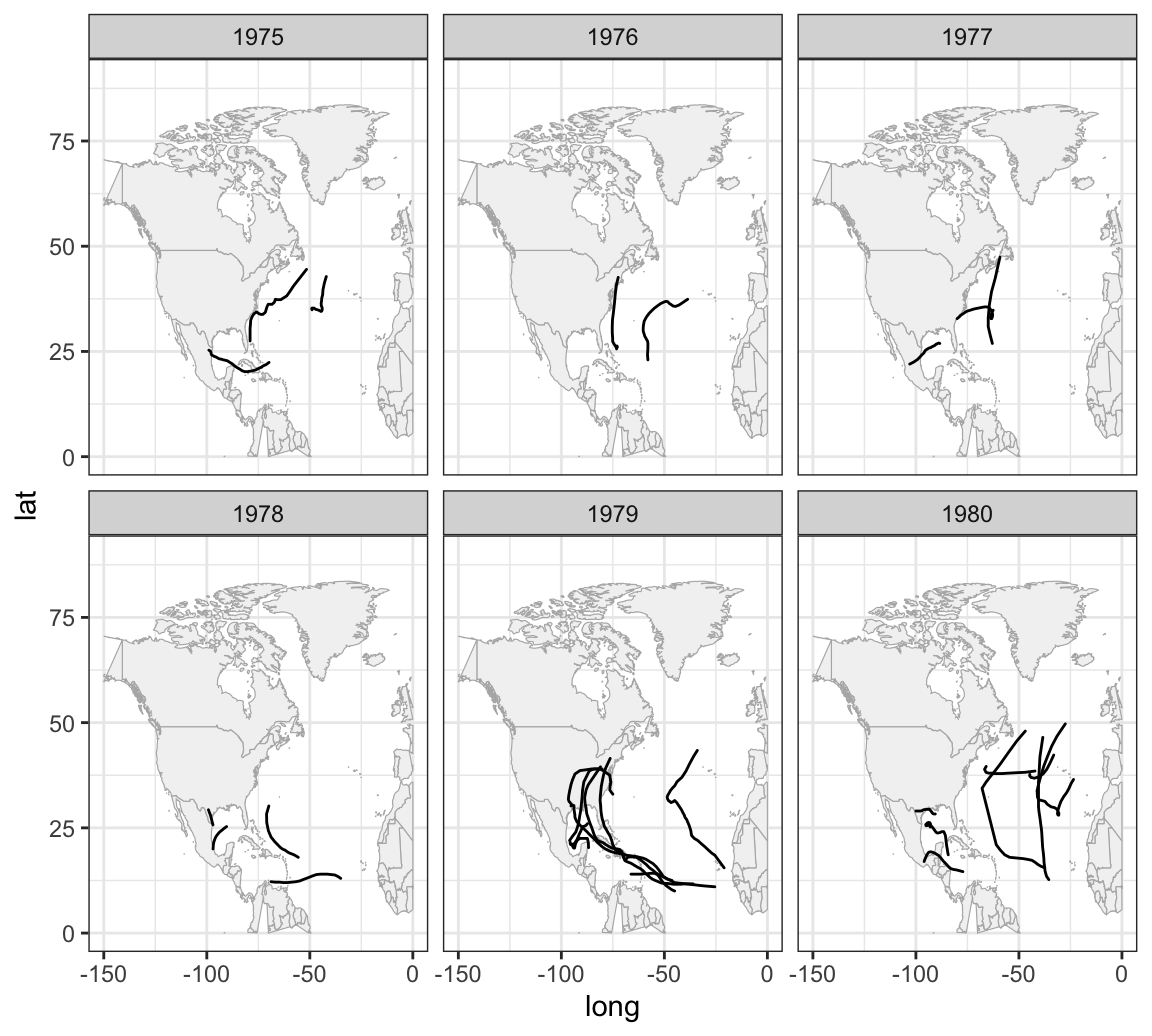11.1.5 Exercises

1) Filter storms in the 1980’s decade (1980 - 1989) and make a plot, with facets by month as well as by year.

• Which year seems to have the largest number of storms?
• Which year seems to have the smallest number of storms?
• Does it seem to be a pattern (e.g. increasing number of storms over years)?

2) Take the previous data, storms in the 1980’s decade, and make a plot, with but this time with facets by month.

• What is the most active month?
• What is the least active month?
• Are there months without any storms?